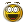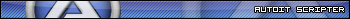# calculation error

Go to solution Solved by jchd,

## Recommended Posts

Hello

In a program, I have to do the addition of several numbers.

But instead of getting 32.04, I get 32.0400000000001

I can reduce this result to 2 digits after the decimal point but why this result, while there are always 2 digits after the decimal point ?

I do not know from where comes the problem, so I've put all the numbers in addition to test.

Thank you

```\$cal = 0.19+0.11+0.4+0.35+0.43+0.27+1.22+0.07+0.04+0.2+6.8+0.04+0.03+0.24+0.62+0.75+0.77+0.18+0.77+0.1+1.35+1.05+0.01+0.01+2.46+5.94+0.19+0.04+2.27+0.02+0.03+0.03+0.03+0.03+0.02+0.02+0.03+0.03+0.03+0.03+0.03+0.03+0.03+0.03+0.03+0.02+0.03+0.03+0.03+0.03+0.03+0.02+0.03+0.03+0.03+0.03+0.03+0.03+0.04+0.03+0.03+0.03+0.03+0.03+0.02+0.02+0.03+0.03+0.03+0.03+0.03+0.03+0.03+0.03+0.03+0.03+0.03+0.03+0.03+0.03+0.03+0.03+0.03+0.03+0.03+0.03+0.03+0.03+0.03+0.03+0.03+0.32+0.17+1.35+0.45+1.05
MsgBox(4096,"",\$cal)```

##### Share on other sites

Hi. use StringFormat

```\$cal = 0.19+0.11+0.4+0.35+0.43+0.27+1.22+0.07+0.04+0.2+6.8+0.04+0.03+0.24+0.62+0.75+0.77+0.18+0.77+0.1+1.35+1.05+0.01+0.01+2.46+5.94+0.19+0.04+2.27+0.02+0.03+0.03+0.03+0.03+0.02+0.02+0.03+0.03+0.03+0.03+0.03+0.03+0.03+0.03+0.03+0.02+0.03+0.03+0.03+0.03+0.03+0.02+0.03+0.03+0.03+0.03+0.03+0.03+0.04+0.03+0.03+0.03+0.03+0.03+0.02+0.02+0.03+0.03+0.03+0.03+0.03+0.03+0.03+0.03+0.03+0.03+0.03+0.03+0.03+0.03+0.03+0.03+0.03+0.03+0.03+0.03+0.03+0.03+0.03+0.03+0.03+0.32+0.17+1.35+0.45+1.05
MsgBox(4096,"",StringFormat("%.2f",\$cal))```

SaludosDanysys.comAutoIt...

##### Share on other sites

• Solution

Floating-point uses binary representation internally, not decimal. Hence rounding errors can accumulate very easily.

This is inherent to IEEE754 floating-point, so AutoIt by itself can do nothing to help. Use Round(), StringFormat() or shift all all your inputs by 10n and perform calculation in integers.

This wonderful site allows debugging and testing regular expressions (many flavors available). An absolute must have in your bookmarks.
RegExp tutorial: enough to get started
PCRE v8.33 regexp documentation latest available release and currently implemented in AutoIt beta.

SQLitespeed is another feature-rich premier SQLite manager (includes import/export). Well worth a try.
SQLite Expert (freeware Personal Edition or payware Pro version) is a very useful SQLite database manager.
An excellent eBook covering almost every aspect of SQLite3: a must-read for anyone doing serious work.
SQL tutorial (covers "generic" SQL, but most of it applies to SQLite as well)
A work-in-progress SQLite3 tutorial. Don't miss other LxyzTHW pages!
SQLite official website with full documentation (may be newer than the SQLite library that comes standard with AutoIt)

##### Share on other sites

Thank you Danyfirex

Yes it works, we can also use ROUND but that I wanted to know is if it was normal or if it was a bug.

Jchd

Thank you for the explanation
Edited by Xerix
##### Share on other sites

You can see the phenomenom by yourself by running this simple script:

```ConsoleWrite(Hex(0.75) & @LF)    ; 3FE8000000000000
ConsoleWrite(Hex(0.3) & @LF)      ; 3FD3333333333333```

Point your browser to this page to better see the breakout of those values and see that 0.3 has an infinite fractional binary expansion which can only be approximated within a 64-bit FP value.

EDIT: while I'm at it, keep in mind that FP has two distinct representations of zero: +0.0 and -0.0

Fortunately AutoIt compares them equal, despite their hex representation differ:

```ConsoleWrite("0.0 = " & Hex(0.0) & @LF)
ConsoleWrite("-0.0 = " & Hex(-0.0) & @LF)
ConsoleWrite("0.0 = -0.0 " & (0.0 = -0.0) & @LF)
ConsoleWrite("-0.0 > 0 " & (-0.0 > 0) & @LF)
ConsoleWrite("-0.0 < 0 " & (-0.0 < 0) & @LF)```
Edited by jchd

This wonderful site allows debugging and testing regular expressions (many flavors available). An absolute must have in your bookmarks.
RegExp tutorial: enough to get started
PCRE v8.33 regexp documentation latest available release and currently implemented in AutoIt beta.

SQLitespeed is another feature-rich premier SQLite manager (includes import/export). Well worth a try.
SQLite Expert (freeware Personal Edition or payware Pro version) is a very useful SQLite database manager.
An excellent eBook covering almost every aspect of SQLite3: a must-read for anyone doing serious work.
SQL tutorial (covers "generic" SQL, but most of it applies to SQLite as well)
A work-in-progress SQLite3 tutorial. Don't miss other LxyzTHW pages!
SQLite official website with full documentation (may be newer than the SQLite library that comes standard with AutoIt)

## Create an account

Register a new account

• ### Recently Browsing   0 members

×

• Wiki

• Back

• #### Beta

• Git
• FAQ
×
• Create New...